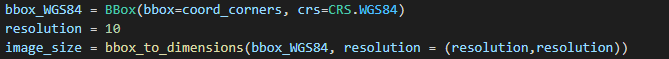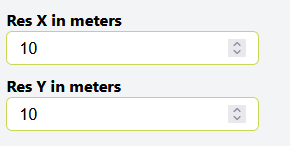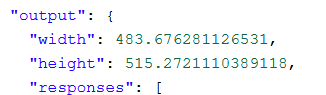# Problem with request builder

Hello,
I dont unterstand why the request builder shows other image sizes, then the function geo_utils.bbox_to_dimensions
So for example, i have these coordinates: coord_corners = [12.787420957808262, 54.00935664138693, 12.861358080662724, 54.055644322669615]
I calculate the image size with a resolution of 10m in x and y direction, like that:And i get for the image_size: 500x500.

So, now i put the same coordinates in the request builder and set the resolution in x and y direction to 10 meter:And get this images sizes:I hope someone can help me! I tried it the whole day and dont get behind it…

Hi Johanna,

The differences appear because both products use a different method for calculating the pixel size of the bounding box.

In the Requests Builder case the size is determined by using the haversine formula (in the case of `x` it returns the maximum size for both the top and bottom boundaries of the bounding box).

In the sh-py package this is done by transforming the bbox to UTM by taking the lower left and upper right vertices, transforming only those and taking resulting pair of points to define the new bbox in UTM, and using that to calculate the pixel size.

In any case the differences on the actual pixel size should be minimal (maybe a bit more accurate on RB).

Let me know any other info needed.

Thanks,

Ignasi Espinosa

When I download the image, I get a pixel size from 10,3 meter x 9,7 meter… So i think i have to live with that?

Hi Johanna,

Using EPSG:4326 and the sh-py bbox_to_dimensions util I think so.

Edit: You can try to use the haversine formula mentioned below to get more accurate results.

Let me know if you need more details.

Ignasi Espinosa

The Problem is now, when i use your code, i get a resolution from 5.88 m x 5,88 m and the image has the size 823x877 Pixel…

You’re right Johanna, sorry for the inconvenience, the above snippet doesn’t work. I will remove it in case other users face the same problem.

In this case, to improve the error you could try to implement the procedure applied on Requests Builder on your own, that is, getting the dimensions by applying Haversine formula on the 4326 bounding box (this will also be an approximation, but it may be more accurate).

Here’s a snippet that may be of use to you:

``````from sentinelhub import CRS, BBox
from math import radians, cos, sin, asin, sqrt

coord_corners = [12.787420957808262, 54.00935664138693, 12.861358080662724, 54.055644322669615]

def haversine(lat1, lon1, lat2, lon2):
"""
Calculate the great circle distance between two points
on the earth (specified in decimal degrees)
"""
lon1, lat1, lon2, lat2 = map(radians, [lon1, lat1, lon2, lat2])

# haversine formula
dlon = lon2 - lon1
dlat = lat2 - lat1
a = sin(dlat/2)**2 + cos(lat1) * cos(lat2) * sin(dlon/2)**2
c = 2 * asin(sqrt(a))
r = 6371
return c * r * 1000

def get_dimensions(bbox, resolution):
xDistance1 = haversine(bbox, bbox, bbox, bbox)
xDistance2 = haversine(bbox, bbox, bbox, bbox)
yDistance = haversine(bbox, bbox, bbox, bbox)
return (max(xDistance1, xDistance2) / resolution, yDistance / resolution)

bbox = BBox(coord_corners, crs= CRS.WGS84)

print(get_dimensions(coord_corners, 10))
``````

This code is just a snippet: the results will also be an approximation and can contain errors

Again, sorry for the inconvience.

Ignasi

First of all, no problem and thanks!
I give it a try, but i have a question. Why do you want as input for the get_dimensions-function a bbox? And you are giving the variable coord_corners, although you calculated bbox?

Hi Johanna,

This is just a quick snippet using the haversine formula from the internet that to get the approximate dimensions. That’s why it uses the bbox as an array of coordinates instead of using an instance of the class `BBox`, since it doesn’t belong to `sentinelhub-py` library.
For making the request you should still use the `BBox` instance as normal, you could try to implement this function to accept a `BBox`, but for demonstrating purposes I think it’s okay.

Ignasi Espinosa

Thank you very much, you helped me a lot…